# Logic Gates

In electronics, Logic gates are the building blocks of every electronic circuit. Logic gates defined as the simple electronic circuits having one or more input and a single output. Their operation varies from one type of logic gate to another. The relationship between input and output follow a certain logical equation.

## What are Logic Gates?

Logic gate can be defined as a simple Boolean function, which means that it has only either of two outputs. Either 1 or 0, high or low, true or false, whatever you can call it. It is the most elementary tool in building a circuit. A logic gate performs a logical operation on input and gives the appropriate output for it.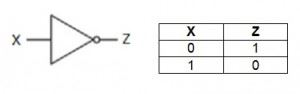This function will have a single input only. It is also called an inverter as it gives the reverse of the input function.

## Types of Logic Gates

A logic gate is a basic building block of an electronic circuit, especially digital circuit, which is used to implement a Boolean function. Given below are the different types of logic gates, their symbols, descriptions and truth tables.

### AND GATE

AND gate is one of the logic gates with two or more inputs and one output. When both the inputs are 1, the output will be 1. The output obtained from AND gate is the dot product of the inputs.

AND gate truth table is given below:

 X Y Z 0 1 0 1 0 0 0 0 0 1 1 1

The AND gate finds application as :

• Enable gate: When the AND gate is used as enable gate, the data is allowed through the channel
• Inhibit gate: When the AND gate is used as inhibit gate, the data is not allowed through the channel

Related Articles:

### OR GATE

If “anyone” of the inputs is 1, the output is 1. The output is 0 only if all inputs are 0. It can have more than 1 input.

Following is the OR gate circuit symbol and truth table: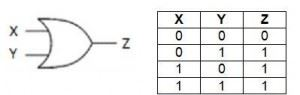There are many real life applications of OR gate in:

• Alarm circuit system for car door

### XOR GATE

It is the ‘Exclusive – OR’ gate. The output is 1 only when one of the inputs is 1. And 0 if the inputs are the same. Following is the XOR gate circuit symbol and truth table: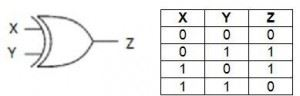There are few applications of  XOR gate like:

### XNOR GATE

It is the ‘Exclusive – NOR’ gate is a combination of XOR gate followed by an inverter. The output is 1 only when both the inputs (even high) are the same and false if the inputs are different. Following is the XNOR gate circuit symbol and truth table: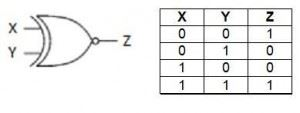There are few applications of  XNOR gate like used in:

• Arithmetic operations
• Parity checker
• Controlled inverter

## What are Universal Gates?

A universal gate is one of the logic gate which uses Boolean function {0,1}. NAND and NOR gates are called universal gates. Following are the reasons why they are called universal gates:

• These gates are used for realizing the binary operations
• The basic logic gates can be derived using universal gates

Universal gates also have following properties:

• These gates are not associative in nature
• These gates are commutative in nature

### NAND GATE

NAND gate is one of the universal gates which is the opposite of AND logic gate. It is a combination of AND and NOT gate and is one of the most commonly used gates.

NAND gate consists of more than one input with single output. To get 1 as the output, all the inputs of the gate should be 0 and to get 0 as the output all the inputs should be 1.

Below is the circuit diagram and truth table of NAND gate: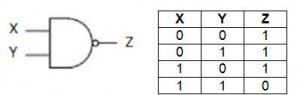The NAND gates find applications in:

• Freezer warning buzzers
• Burglar alarm
• In automated circuit

### NOR GATE

It is the NOT – OR gate. The inverter bubble reverses the output of the OR gate logic giving 1 as the output only if all inputs are 0. It can have more than 1 input. The circuit diagram and truth table is given below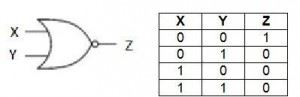Applications of NOR gate are:

• They are used in combination of circuits like multipliers, ripple carry address, half and full wave multipliers.
• They are used in the construction of sequential circuits.

Summary

Following is the table explaining the logical gate formulas:

 Logical gate Boolean notation AND X.Y NAND $\bar{X.Y}$ OR X+Y NOR $\bar{X+Y}$ XOR $X\bigoplus Y$ XNOR $\bar{X\bigoplus Y}$

## FAQs on Logic Gates

Q1. When will the output of AND gate be high, with inputs X and Y and output Z?

Ans: For the output to be high in an AND gate, following are the conditions:

X = 1

Y = 1

Then, the output Z = 1.